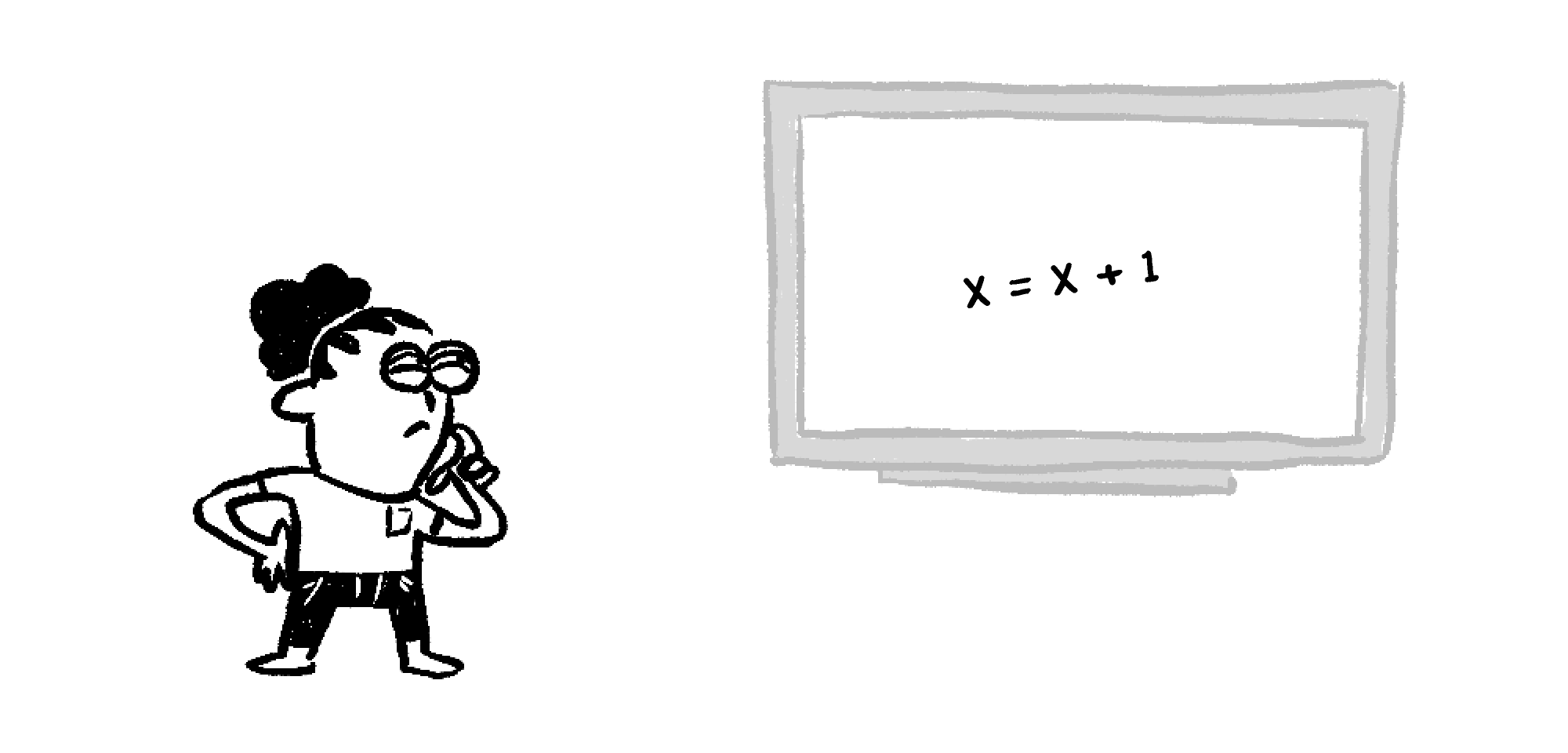Back

## What's in a Variable?

Variables in math are representations of a single, unknown quantity. “Variables” in computer science aren’t one single thing, and they’re not unknown. Without batting an eyelid, programmers will write things like this:This is clearly absurd! There’s no $x$ such that $x = x + 1$. What's going on in the brains of programmers?In computer science, an assignable variable is a place in memory where a program can store a value. The same memory location can store different values at different times as a program runs.

A program accesses an assignable variable in two distinct ways:

• it may write (i.e. store) a value, or
• it may read (i.e. retrieve) a value.

The program is instructed to both write and read in the single 'sentence'which is valid in many programming languages, including C and Python. Unpacking its meaning in plain English, this instruction means the following:

Write a new value at location x that is one more than the current value stored at location x, erasing the value that was in that location before.

That is, when x occurs to the left of the = sign, it is being accessed as a location for writing information, whereas the x to the right of = is being accessed as a location for reading information. The right side of the equation must be executed first for this to make sense.

To avoid confusion, here at Brilliant we will use pseudocode to express algorithms, and we will express the above operation as follows:Whenever an assignable variable's name is preceded by the word set, it means that the program is writing to the location. In all other situations, the program is reading from the location.

Consider the following program:

Mathematically, it looks like after the program is run, x and y should store the same value — the sum x+y. But that is not how it works. The program executes instructions in order from top to bottom. Watch the program run with x initially set to $1$ and y initially set to $3:$

In the first set instruction, the program reads the values stored at x and y $(1$ and $3)$ and adds them to get $4.$ The new value $(4)$ is stored at location x, overwriting the $1$ that was there before.

In the second set instruction, the program again reads the values stored at x and y. This time, they are $4$ and $3$, which sum to $7$, and this new sum is stored at location y.

At the end of the program, x contains $4$ and y contains $7$ — definitely different values. But because the program runs from top to bottom, the order of the instructions can also affect the outcome.

In the program below, try dragging the tiles into the program to see how the result changes:

Because the variable in the first instruction appears in the assignment rule of the second variable, you should see different values for x and y when you assign y first. You ain't seen a mathematical variable do anything like that!

# Today's Challenge

Rearrange the tiles in the program below. Initially (before the four-tile program runs), num contains $0.$ After the program runs, num may contain a $1,$ $2,$ or $3$ digit number.

$225$ is the largest possible output from this program. What is the largest two-digit number you can make by rearranging the tiles?

See if you can figure out the answer without manually trying every combination of tiles. $\\[1.2em]$We are sunsetting our community features by July 2, 2021. Learn more here.
×

Problem Loading...

Note Loading...

Set Loading...